Processing ......FreeComputerBooks.com Links to Free Computer, Mathematics, Technical Books all over the World

Computability Theory: Introduction to Recursion Theory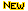Top Free Programming Books - 100% Free or Open Source!
• Title Computability Theory: Introduction to Recursion Theory
• Author(s) Karl-Heinz Zimmermann, Frank Stephan, Jaap van Oosten, Jeremy Avigad, et al
• Publisher: Hamburg University of Technology, National University of Singapore, Utrecht University, et al
• Paperback N/A
• eBook PDF
• Language: English
• ISBN-10: N/A
• ISBN-13: N/A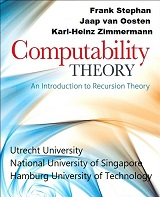Book Description

Computability Theory originated with the seminal work of Gödel, Church, Turing, Kleene and Post in the 1930s. This theory includes a wide spectrum of topics, such as the theory of reducibilities and their degree structures, computably enumerable sets and their automorphisms, and subrecursive hierarchy classifications.

Recent work in computability theory has focused on Turing definability and promises to have far-reaching mathematical, scientific, and philosophical consequences.

These texts provide concise, comprehensive, and authoritative introduction to contemporary computability theory, techniques, and results. The basic concepts and techniques of computability theory are placed in their historical, philosophical and logical context.

The texts include both the standard material for a first course in computability and more advanced looks at degree structures, forcing, priority methods, and determinacy. They also explore a variety of computability applications to mathematics and science.

These are invaluable texts, references, and guides to the direction of current research in the field. Nowhere else will you find the techniques and results of this beautiful and basic subject brought alive in such an approachable way.

• N/A

Reviews, Ratings, and Recommendations: Related Book Categories: Read and Download Links:Similar Books:
•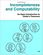Incompleteness and Computability: Gödel's Theorems

This book is an introduction to metamathematics and Gödel's Theorems. It covers recursive function theory, arithmetization of syntax, the first and second incompleteness theorem, models of arithmetic, second-order logic, and the lambda calculus.

•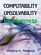Computability, Unsolvability, Randomness (Stephen G. Simpson)

The author exposits Turing's 1936 theory of computability and unsolvability, as subsequently developed by Kleene and Post. This theory is of the essence in theoretical computer science and in the study of unsolvable mathematical problems.

•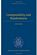Computability and Randomness (Andre Nies)

The complexity and the randomness aspect of a set of natural numbers are closely related. This book includes a detailed treatment of Turing's theory of computability and unsolvability as subsequently developed by Kleene, Post, Friedberg, etc.

•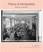Theory of Computation, Making Connections (Jim Hefferon)

The book is geared toward those who thirst for computation theory knowledge. To cater to the demands of a wide range of people, the principles in this book are explained in a way that is easy to understand, digest and apply in the upcoming career.

•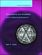Computability and Complexity: From a Programming Perspective

This book is an introduction to the basic concepts of computability, complex, and the theory of programming languages. Its goal is to build a bridge between computability and complexity theory and other areas of computer science, especially programming.

•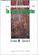Introduction to Theory of Computation (Eitan M. Gurari)

This book explores some of the more important terminologies and questions concerning programs, computers, problems, and computation. The exploration reduces in many cases to a study of mathematical theories, such as those of automata and formal languages.

•Models of Computation: Exploring the Power of Computing

It covers the traditional topics of formal languages, automata and complexity classes, as well as an introduction to the more modern topics of space-time tradeoffs, memory hierarchies, parallel computation, the VLSI model, and circuit complexity.

•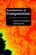Foundations of Computation (Carol Critchlow, et al)

It includes topics from discrete mathematics, automata theory, formal language theory, and the theory of computation, along with practical applications to computer science. The course has no prerequisites other than introductory computer programming.

Book Categories
 :All CategoriesTop BooksRecent BooksMiscellaneous BooksComputer LanguagesComputer ScienceData Science/DatabasesElectrical EngineeringJava and Java EE (J2EE)Linux and UnixMathematicsMicrosoft and .NETMobile ComputingNetworking and CommunicationsSoftware EngineeringSpecial TopicsWeb Programming
Other Categories